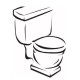# SPOJ: Absurd Prices (6803)

Just writing on a SPOJ problem I worked on recently. It’s called Absurd Prices, code name ABSURD.

In this problem, when given an integer representing an item’s price in cents, our program has to determine whether the price is absurd or not.

There is an algorithm to calculate the absurdity of an integer: the higher the absurdity, the more absurd it is. An integer$c$ is considered absurd when within the range$[0.95c,1.05c]$ there is an integer with a lower absurdity.

To find the absurdity of a price:

1. Remove all the trailing 0’s from it
2. Count the length of the remaining number
3. The absurdity is twice the length
4. If the number (with trailing zeroes removed) ends with 5, subtract 1 from the absurdity

As a result of these rules, prices with a lot of trailing zeroes, like 3500000, would have a very low absurdity, while those without trailing zeroes, like 7999999, would have a much higher absurdity.

A brute force approach seemed at first to be the way to go. This is because the range,$[0.95c,1.05c]$ isn’t too big and it isn’t a big deal to simply calculate the absurdity of all of them, and mark it as absurd when any of the integers in the range has a lower absurdity.

However, the time limit is very strict for this problem. Even when my program only checked 50 random numbers in the range, the system returned with TLE (Time Limit Exceeded)!

Fortunately there is a better way of solving the problem.

Given a range, it is easy to find the least absurd number in the range. For example, if our minimum and maximum bounds are 6655 and 7799 respectively, it’s easy to see that the least absurd number in that range is 7000, simply because it has the most trailing zeroes.

The algorithm that we can use to find the least absurd number in a range (defined by two integers) is follows:

1. In their decimal expansions, compare the first characters, then the second characters, etc, until the$n^{th}$ character is different.
2. The minimum absurdity is$2n$.
3. If the$n^{th}$ character of the smaller number is less than 5, and the$n^{th}$ character of the larger number is five or greater, subtract 1 from the minimum absurdity.

```-- Integer with trailing zeroes removed
removeZeroes x = head . filter (\n -> n `mod` 10 > 0) \$
iterate (\n -> n `div` 10) x

-- Calculuate the absurdity of a price
absurdity x = let
r = removeZeroes x
d = fromIntegral . length \$ show r
l = r `mod` 10
in if l == 5 then 2*d-1 else 2*d

-- The minimum possible absurdity in a given range
minabsd a b | a == b = absurdity a
minabsd a b = let
c = show a; d = show b
sm = fromIntegral . length . takeWhile (uncurry (==)) \$ zip c d
lst = (c!!sm,d!!sm)
offset = if fst lst < '5' && snd lst >= '5' then 1 else 0
in minimum [absurdity a, (2 * (sm + 1) - offset), absurdity b]

absurd x = let
r1 = ceiling \$ 0.95*(realToFrac x)
r2 = floor \$ 1.05*(realToFrac x)
absx = absurdity x
in absx > minabsd r1 r2

main = interact run where
as s = let k = read s in if absurd k then "absurd" else "not absurd"
run = unlines . map as . tail . lines
```

## 4 thoughts on “SPOJ: Absurd Prices (6803)”

1.raj says:

as you said that we compare characters in their decimal representation, so what if the lengths are not equal?

Like

2.luckytoilet says:

Oh I think that’s pretty trivial. If you have xxxxx and xxxxxx then the lowest in the bound is 100000.

Like

3.raj says:

sorry, but didn’t get you,is the value always 100000 for any difference in length? E.g, [950000,1050000]

Like

4.luckytoilet says:

Oh no. In that case your minimum value should be 1000000 (absurdity value of 2).

Edit- Strictly speaking, my implementation of minabsd isn’t correct (it crashes for 9 and 99 for instance). This turns out to be inconsequential as these inputs never actually occur. Now when they don’t start with the same leading number, sm evaluates to 0 which gives the correct answer.

Like